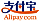Copertina di Sobolev space
Titolo del libro:

# Sobolev space

Betascript Publishing (16.09.2013 )eleggibile a buono
ISBN-13:

### 978-613-0-32039-3

ISBN-10:
6130320396
EAN:
9786130320393
Lingua del libro:
Inglese
Risvolto di copertina:
Please note that the content of this book primarily consists of articles available from Wikipedia or other free sources online. In mathematics, a Sobolev space is a vector space of functions equipped with a norm that is a combination of Lp norms of the function itself as well as its derivatives up to a given order. The derivatives are understood in a suitable weak sense to make the space complete, thus a Banach space. Intuitively, a Sobolev space is a Banach space, and in some cases a Hilbert space, of functions with sufficiently many derivatives for some application domain, such as partial differential equations, and equipped with a norm that measures both the size and smoothness of a function.
Casa editrice:
Betascript Publishing
Sito Web:
https://www.betascript-publishing.com/
A cura di:
Lambert M. Surhone, Mariam T. Tennoe, Susan F. Henssonow
Numero di pagine:
160
Pubblicato il:
16.09.2013
Giacenza di magazzino:
Disponibile
categoria:
Geoscienze
Prezzo:
49,00 €
Parole chiave:
Mathematics, Normed Vector Space, Weak Derivative, Banach space, Hilbert Space, Partial Differential Equation, Mathematician### Categorie